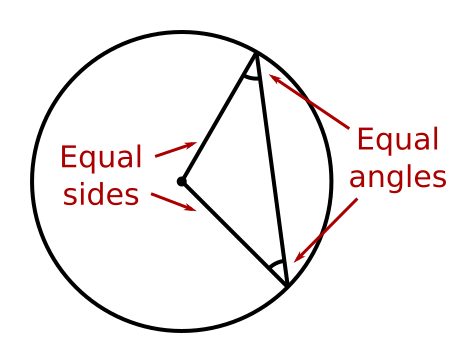# Two radii form an isosceles triangle

Martin McBride
2020-08-16

A triangle formed by two radii of a circle is an isosceles triangle.Since it is a isosceles triangle, it follows that the angles between the two radii and the chord that forms the third side of the triangle are also equal.

This theorem is covered in this video on circle theorems:

## Proof

Two sides of the triangle are radii of the circle, and therefore have the same length. By definition, any triangle with two equal sides is an isosceles triangle.